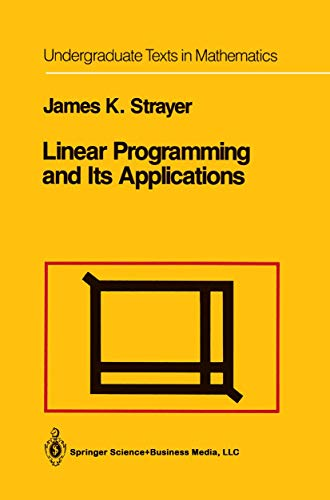• Free Shipping on all orders in Australia
• Over 7 million books in stock
• Proud to be B-Corp
• We aim to be carbon neutral by 2022
• Over 120,000 Trustpilot reviews
Item 1 of 0# Linear Programming and Its Applications by James K. Strayer

Condition - New
\$130.89
Only 2 left

## Summary

Linear Programming and Its Applications is intended for a first course in linear programming, preferably in the sophomore or junior year of the typical undergraduate curriculum.

## Linear Programming and Its Applications Summary

### Linear Programming and Its Applications by James K. Strayer

Linear Programming and Its Applications is intended for a first course in linear programming, preferably in the sophomore or junior year of the typical undergraduate curriculum. The emphasis throughout the book is on linear programming skills via the algorithmic solution of small-scale problems, both in the general sense and in the specific applications where these problems naturally occur. The book arose from lecture notes prepared during the years 1985-1987 while I was a graduate assistant in the Department of Mathematics at The Pennsylvania State University. I used a preliminary draft in a Methods of Management Science class in the spring semester of 1988 at Lock Haven University. Having been extensively tried and tested in the classroom at various stages of its development, the book reflects many modifications either suggested directly by students or deemed appropriate from responses by students in the classroom setting. My primary aim in writing the book was to address common errors and difficulties as clearly and effectively as I could.

0 Introduction.- I: Linear Programming.- 1 Geometric Linear Programming.- 0. Introduction.- 1. Two Examples: Profit Maximization and Cost Minimization.- 2. Canonical Forms for Linear Programming Problems.- 3. Polyhedral Convex Sets.- 4. The Two Examples Revisited.- 5. A Geometric Method for Linear Programming.- 6. Concluding Remarks.- Exercises.- 2 The Simplex Algorithm.- 0. Introduction.- 1. Canonical Slack Forms for Linear Programming Problems; Tucker Tableaus.- 2. An Example: Profit Maximization.- 3. The Pivot Transformation.- 4. An Example: Cost Minimization.- 5. The Simplex Algorithm for Maximum Basic Feasible Tableaus.- 6. The Simplex Algorithm for Maximum Tableaus.- 7. Negative Transposition; The Simplex Algorithm for Minimum Tableaus.- 8. Cycling.- 9. Concluding Remarks.- Exercises.- 3 Noncanonical Linear Programming Problems.- 0. Introduction.- 1. Unconstrained Variables.- 2. Equations of Constraint.- 3. Concluding Remarks.- Exercises.- 4 Duality Theory.- 0. Introduction.- 1. Duality in Canonical Tableaus.- 2. The Dual Simplex Algorithm.- 3. Matrix Formulation of Canonical Tableaus.- 4. The Duality Equation.- 5. The Duality Theorem.- 6. Duality in Noncanonical Tableaus.- 7. Concluding Remarks.- Exercises.- II: Applications.- 5 Matrix Games.- 0. Introduction.- 1. An Example; Two-Person Zero-Sum Matrix Games.- 2. Linear Programming Formulation of Matrix Games.- 3. The Von Neumann Minimax Theorem.- 4. The Example Revisited.- 5. Two More Examples.- 6. Concluding Remarks.- Exercises.- 6 Transportation and Assignment Problems.- 0. Introduction.- 1. An Example; The Balanced Transportation Problem.- 2. The Vogel Advanced-Start Method (VAM).- 3. The Transportation Algorithm.- 4. Another Example.- 5. Unbalanced Transportation Problems.- 6. The Assignment Problem.- 7. Concluding Remarks.- Exercises.- 7 Network-Flow Problems.- 0. Introduction.- 1. Graph-Theoretic Preliminaries.- 2. The Maximal-Flow Network Problem.- 3. The Max-Flow Min-Cut Theorem; The Maximal-Flow Algorithm.- 4. The Shortest-Path Network Problem.- 5. The Minimal-Cost-Flow Network Problem.- 6. Transportation and Assignment Problems Revisited.- 7. Concluding Remarks.- Exercises.- APPENDIX A Matrix Algebra.- APPENDIX B Probability.- Answers to Selected Exercises.

NLS9781461269823
9781461269823
1461269822
Linear Programming and Its Applications by James K. Strayer
New
Paperback
Springer-Verlag New York Inc.
2012-09-30
266
N/A
Book picture is for illustrative purposes only, actual binding, cover or edition may vary.
This is a new book - be the first to read this copy. With untouched pages and a perfect binding, your brand new copy is ready to be opened for the first time